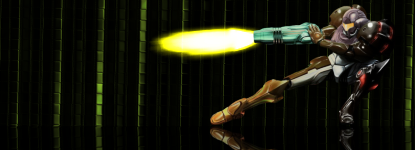# Outdated example, need some help

## Recommended Posts

I'm trying to write effects in my spare time, very simple ones to get me started.

I've been using the ones provided on boltbait.com, but some of the code is outdated

Recently I've been trying to make lines at various angles

This is the example provided

```// submenu: Render
#region UICode
double Amount1 = 45; // [-180,180] Angle
#endregion

void Render(Surface dst, Surface src, Rectangle rect)
{
int w = dst.Width, h = dst.Height;
for (int y = rect.Top; y < rect.Bottom; y++)
{
for (int x = rect.Left; x < rect.Right; x++)
{
int u = 2 * x - w, v = 2 * y - h;
dst[x, y] = ColorFunc(
Math.Sqrt(u * u + v * v)+Amount1,
Math.Atan2(v, u)
);
}
}
}

ColorBgra ColorFunc(double r, double t)
{
r /= 100;
return ColorBgra.FromBgr(
Utility.ClampToByte(128+127*Math.Sin(r+t)),
Utility.ClampToByte(128+127*Math.Sin(Math.PI/2+r+t)),
Utility.ClampToByte(128+127*Math.Sin(Math.PI+r+t))
);
}```

Utility.ClampToByte is no longer used by PDN apparently, so how do I rewrite the last lines?

I tried on my own, but it caused several errors with converting double to int values (which I also tried to solve)##### Share on other sites

Just write your own Clamp function, it should look something like this. (I haven't tested this yet.)

```byte Clamp2Byte(int value)
{
if (value > 255)
{
return 255;
}
else if (value < 0)
{
return 0;
}
else
{
return (byte)value;
}
}

```

EDIT: Sorry for the wierd formating. I tried to fix it but it won't format correctly.##### Share on other sites

Just write your own Clamp function

Shorter version (not tested either):

```static byte ClampToByte(int input)
{
return (byte)Math.Max(Math.Min(input, 255), 0);
}
```

##### Share on other sites

Shorter version (not tested either):

```static byte ClampToByte(int input)
{
return (byte)Math.Max(Math.Min(input, 0), 255);
}
```

Switch the max & min. I think.... really tired........

zzzzzzzzzzzzzzzz

http://i.imgur.com/xZYt6wl.png

ambigram signature by Kemaru

[i write plugins and stuff]

If you like a post, upvote it!

##### Share on other sites

Umm, guys, Utility.ClampToByte's obsolete tag also includes a message telling you precisely what to do instead. It says, "Use Int32Util.ClampToByte() instead."

Ergo, my guess is that Int32Util.ClampToByte() might be useful.

The Paint.NET Blog: https://blog.getpaint.net/

Donations are always appreciated! https://www.getpaint.net/donate.html

##### Share on other sites

Is the obsolete attribute's message not showing up in CodeLab?

The Paint.NET Blog: https://blog.getpaint.net/

Donations are always appreciated! https://www.getpaint.net/donate.html

##### Share on other sites

The error it gives is:

"'PaintDotNet.Utility.ClampToByte(int)' is obsolete: 'Use the ClampToByte extension method instead' (CS0619)"

It does say anything about "Int32Util.ClampToByte()".##### Share on other sites

Umm, guys, Utility.ClampToByte's obsolete tag also includes a message telling you precisely what to do instead. It says, "Use Int32Util.ClampToByte() instead."

Ergo, my guess is that Int32Util.ClampToByte() might be useful.

That's what I tried first, and then conversion errors arose.

It's rather probable that I just don't know what I'm doing. I have virtually no proper knowledge in C#.

I started coding on a TI-83, then started teaching myself C#. When college starts I'll get some real classes on this, but so far I only know what I've learned from online tutorials##### Share on other sites

Ahh I must have changed the comment in the v4.0 code base then. The Utility class is being phased out, its functionality being moved into various *Util and *Extensions static classes.

Extension methods are easy to use too.

```int x = ...;
byte y = x.ClampToByte();

byte z = (int)(some expression).ClampToByte();```

The Paint.NET Blog: https://blog.getpaint.net/

Donations are always appreciated! https://www.getpaint.net/donate.html

##### Share on other sites

```ColorBgra ColorFunc(double r, double t)
{
r /= 100;
return ColorBgra.FromBgra(

(int)(128+127*Math.Sin(r+t)).ClampToByte(),
(int)(128+127*Math.Sin(r+t)).ClampToByte(),
(int)(128+127*Math.Sin(Math.PI+r+t)).ClampToByte()

);
}
```

Like that?

" 'double' does not contain a definition for 'ClampToByte' "

Does that even make sense?...##### Share on other sites

That's because the extension method is being applied before the typecast operator is.

`(int)(some double expression).ExtensionMethod();`

is equivalent to

`(int)((some double expression).ExtensionMethod());`

Notice the placement of parenthesis.

The Paint.NET Blog: https://blog.getpaint.net/

Donations are always appreciated! https://www.getpaint.net/donate.html

##### Share on other sites

```ColorBgra ColorFunc(double r, double t)
{
r /= 100;
return ColorBgra.FromBgra(

(int)(128+127*Math.Sin(r+t)).ClampToByte(),
(int)(128+127*Math.Sin(r+t)).ClampToByte(),
(int)(128+127*Math.Sin(Math.PI+r+t)).ClampToByte()

);
}
```

Like that?

" 'double' does not contain a definition for 'ClampToByte' "

Does that even make sense?...

It makes perfect sense; you're trying to call a method called "ClampToByte" on a double, and it doesn't have one. You need to cast to an int first, since that's what the extension is defined for.

((int)(128+127*Math.Sin(r+t))).ClampToByte()

Note how the .ClampToByte is now being called on the int-casted expression, instead of just "(128+127*Math.Sin(r+t))" which is a double.

http://i.imgur.com/xZYt6wl.png

ambigram signature by Kemaru

[i write plugins and stuff]

If you like a post, upvote it!

##### Share on other sites

Oh so, (int) defines it as an integer, and without placing parenthesis around it like this

((int)(128+127*Math.Sin(r+t))).ClampToByte()

It's left out of the ClampToByte() Method?

I didn't realize the ClampToByte() was a method. It's rather obvious now, I just tend to overlook some of the more obvious things like this haha.

So now it says int doesn't have a definition in ClampToByte

Ugh, I hate being so ignorant and useless## Join the conversation

You can post now and register later. If you have an account, sign in now to post with your account.×   Pasted as rich text.   Paste as plain text instead

Only 75 emoji are allowed.

×   Your previous content has been restored.   Clear editor

×   You cannot paste images directly. Upload or insert images from URL.

×
×